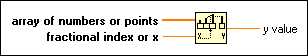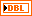# Interpolate 1D Array Function

## LabVIEW 2018 Help

Edition Date: March 2018
Part Number: 371361R-01
View Product InfoLabVIEW 2016 HelpLabVIEW 2017 HelpLabVIEW 2018 HelpLabVIEW 2019 HelpLabVIEW 2020 Help

Owning Palette: Array Functions

Requires: Base Development System

Linearly interpolates a decimal y value from an array of numbers or points using a fractional index or x value.

The connector pane displays the default data types for this polymorphic function.array of numbers or points can be an array of numbers or an array of points where each point is a cluster of x and y coordinates. If this input is an array of points, the function uses the first element in the cluster (x) to obtain a fractional index by linear interpolation. The function then uses this fractional index to compute the output y value from the second cluster element (y).fractional index or x is the index or x-value at which the function should return a y-value. For example, if array of numbers or points contains two double-precision, floating-point numeric values, 5 and 7, and fractional index or x is set to 0.5, the function returns 6.0, which is halfway between the values at elements 0 and 1. If array of numbers or points contains an array of data point sets, the function returns the linearly interpolated y value at the x-value corresponding to fractional index or x. For example, if the array contains two points, (3,7) and (5,9), and fractional index or x is set to 3.5, the function returns 7.5. fractional index or x does not interpolate beyond the bounds of an array or data point set. For example, if the parameter is set lower than the first element or x-value in an array, the function returns the value of the first element or the y-value of the first data point. Similarly, if the parameter is set too high, the function returns the value in the final element or the final y-value. fractional index or x must be located directly on a point or between two points for the function to work correctly.y value is the interpolated value of the element at the fractional index or the interpolated y-value of the fractional data point, in array of numbers or points.

## Interpolate 1D Array Details

You can wire an array of numeric values or an array of data point sets to this function. If you wire an array of numeric values, the function interprets fractional index or x as a reference to the array elements. If you wire an array of data point sets, the function interprets fractional index or x as a reference to the x-value elements within each data point set.Note  If you wire an array of data points to this function, the data points must be sorted by increasing x-value.